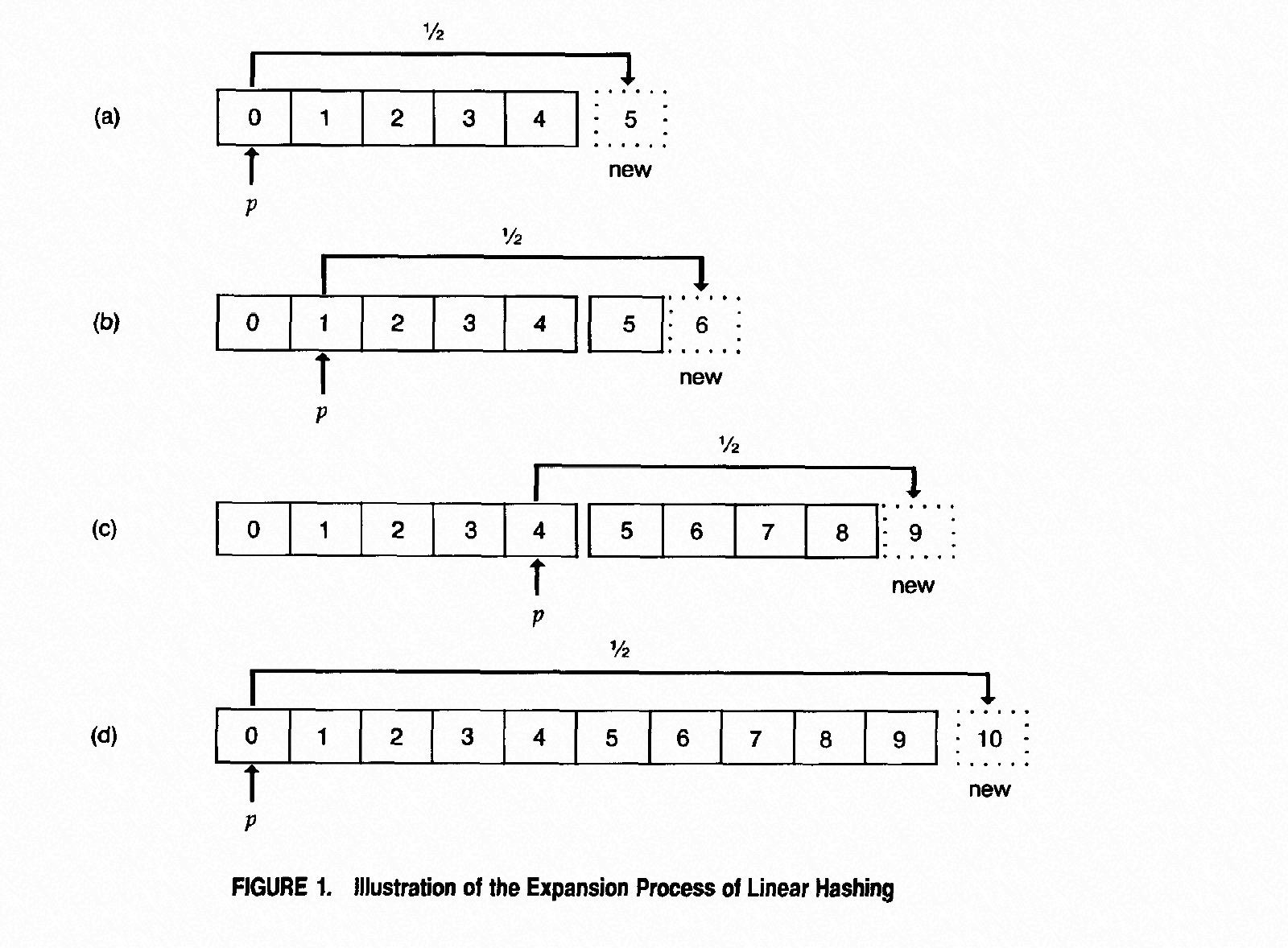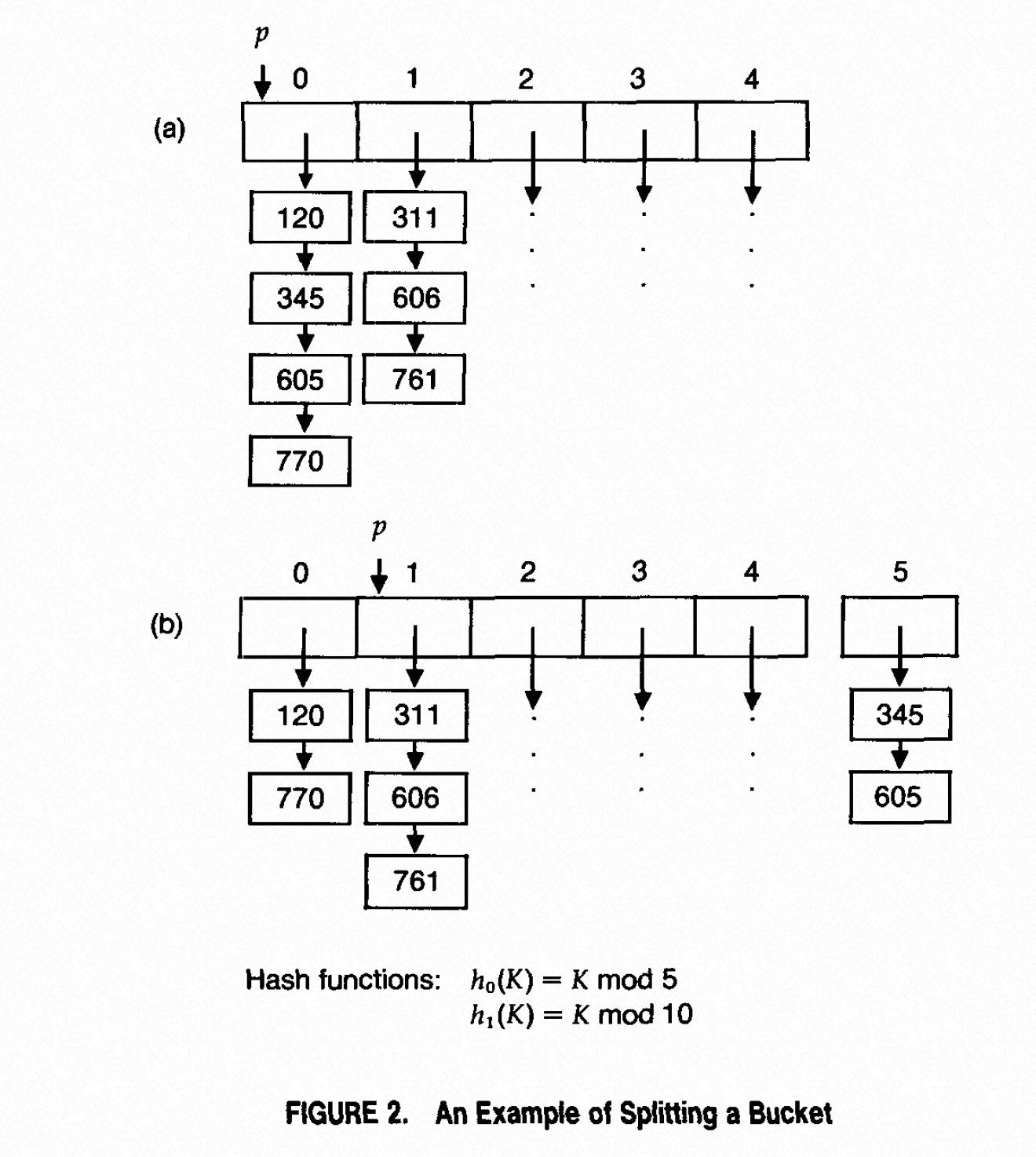### Dynamic hash tables

Communications of the ACM, April 1988

https://dl.acm.org/doi/10.1145/42404.42410

#### Linear Hashing• L: 哈希表 bucket 数目翻倍的次数（L >= 0）
• p: 指向下一个要分离 bucket （0 <= p <= N x 2^L，其中 N 是哈希表初始 bucket 个数）

hj(K) = g(K) mod(N x 2^j), j=0,1,...``````p := p + 1;
if p = N x 2^L then begin
L := L + 1;
p := 0;
end;
``````

``````addr := hL(K);